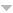# Browse & Filter

For page specific messages
For page author info
3564 records found.
Operations
Title+Summary Name Date### [NOTES/EM-02014] Flux --- Example of a point charge

Node id: 5961page

The flux of the electric field of a point charge placed at the centre  of a sphere is explicitly computed and shown to be

$\text{Flux} = \frac{q}{\epsilon_0 }$

23-10-18 20:10:01 n

### [NOTES/EM-02012] Dipole in Uniform Electric Field

Node id: 5957page

When a dipole is placed in a uniform electric field, it experiences a torque  given by $$\vec \tau= \vec p \times \vec E$$.

23-10-18 19:10:38 n

### [NOTES/EM-02010] Gauss Law and Use of Symmetry

Node id: 5956page

Gauss law aloe is not sufficient to determine the electric field for a given system.To determine electric field using Gauss law the symmetry of problem plays an important role by determining the direction of the electric field in given problem.

23-10-18 19:10:36 n

### [NOTES/EM-03008]-Maxwell's Second Equations from Coulomb's Law

Node id: 5645page

Maxwell's equation, $$\nabla \times \vec{E}=0$$, can be easily proved by direct computation of curl of electric field of a point charge and appealing to the superposition principle.

23-10-18 15:10:52 n

### [NOTES/EM-03007]-Work done in field of a point charge

Node id: 5644page

We discuss the path independence of the work done by static electric field. This leads to, as in mechanics, introduction of the electric potential. An expression of the electric potential is derived by an explicit computation of work done by on a unit positive charge by the electric field of a point charge $$q$$. For an arbitrary distribution of charges, the electric potential is obtained by making use of the superposition principle.

23-10-18 14:10:17 n

### [NOTES/EM-03006]-Electrostatic Energy of a Uniformly Charged Solid Sphere

Node id: 5643page

The electrostatic energy of a uniformly charged solid sphere is computed by computing the energy required to bring infinitesimal quantities and filling up the sphere.

23-10-18 13:10:39 n

### [NOTES/EM-03005]-Multipole Expansion of Potential

Node id: 5642page

The large distance expansion of potential due to a localized charge distribution is obtained. The first three terms receiving contributions from the monopole, the dipole moment and the quadrupole moment are explicitly displayed.  Important properties of dipole and quadrupole moment are discussed.

23-10-18 08:10:38 n

### [NOTES/EM-03003]-Maxwell's Equations from Coulomb's Law

Node id: 5637page

Starting with the Gauss law and using divergence theorem of vector calculus we derive Maxwell's first equation $\nabla\cdot \vec{E}= \rho/\epsilon_0$.

23-10-18 08:10:34 n

### [NOTES/EM-03002]-Electrostatic Energy

Node id: 5635page

Expressions for electrostatic energy of system of point charges is derived.

23-10-18 08:10:36 n

### [NOTES/EM-03004]-The Electric Stress Tensor

Node id: 5641page

An expression for the electric stress tensor is derived for a charge distribution in a volume $$V$$. The surface integral of the stress  tensor gives the total electric force on the  charge in the volume $$V$$.

23-10-17 14:10:45 n

### [NOTES/EM-03001]-Computation of Electric Potential

Node id: 5634page

The curl free nature of the electric field in electrostatics implies existence of a potential,$$\phi(\vec(r))$$, from which the electric field can be derived as $$\vec{E}=-\nabla \phi$$. The potential at a point is just the work done in moving a unit point charge from infinity to its current position.

23-10-17 14:10:31 n

### [NOTES/EM-02015] Proof of curl free nature of $$\vec E$$

Node id: 5960page

Starting from Coulomb's law a proof is given that the electric field of a system of point charges obeys the Maxwell's equation.
$\nabla \times \vec E =0$

23-10-12 19:10:59 n

### [NOTE/EM-02014] Flux of Feld of a Point Charge

Node id: 5959article
23-10-12 19:10:58 n

### [NOTES/EM-02013] Solid Angle

Node id: 5958page

In this section the concept of solid angle is defined as a generalization of angle in plane geometry

23-10-12 17:10:14 n

### [NOTES/EM-02009] Line Integrals In Physics

Node id: 5955page

A few examples of problems are given from electromagnetic theory and other areas of physics are given  in which the line integral appears.

23-10-12 17:10:01 n

### [NOTES/EM-02007]-Maxwell's Equations for Electrostatics-I

Node id: 5579page

The Gauss law of electrostatics follows from the Coulomb’s law for a point charge and superposition principle. The Gauss law along with the Gauss divergence theorem of vector calculus imply Maxwell’s first equation $$\nabla\cdot\bar{E}=\rho/\epsilon_0$$ for electrostatics

23-10-10 20:10:14 n

### [NOTES/EM-02008]-Maxwell's Equations for Electrostatics-II

Node id: 5580page

The Gauss law of electrostatics follows from the Coulomb's law for a point charge and superposition principle. The Gauss law along with the Gauss divergence theorem of vector calculus imply Maxwell's first equation $\nabla\cdot\bar{E}=\frac{\rho}{\epsilon_0}.$ for electrostatics.

23-10-10 20:10:28 n

### [NOTES/EM-02006]-Proof of Gauss Law

Node id: 5578page

The Gauss law of electrostatics follows from the Coulomb’s law for a point charge and superposition principle. The proof given here follows Feynman’s lectures. It makes use of two important features of the electric field due to a point charge. These are
(i) the magnitude of the field obeying the inverse square law, and
(ii) radial direction of the electric field of a point charge. The above two properties are essential to the proof. Gauss law will not hold for hypothetical field, not having both the properties.

23-10-09 04:10:51 n

### [NOTES/EM-02004]-Applications of Gauss Law

Node id: 5570page

Gauss law is applied to compute the electric field for several systems, see the Table of Contents for details. The symmetry of the problem is used in a nontrivial manner to arrive at the answers for the electric field.

23-10-07 05:10:29 n

### [NOTES/EM-02003]-Electric Field due to Continuous Charge Distributions

Node id: 5568page

Electric field due to several charge distributions, listed in the table of contents, is computed using Coulomb’s law.

23-10-07 05:10:47 n

X